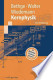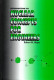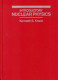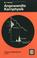Fundamentals of Nuclear Physics

Course Description

External and internal properties of the atomic nucleus (charge, mass, size, binding energy, spin, electric and magnetic moments). Models of the atomic nucleus (liquid drop model, shell model). Nuclear collisions and reactions (conservation laws, cross sections, fission, fusion). Nuclear decays and radioctivity (radioactive decay law, radioactive decay chains, alpha-decay, beta-decay, gamma decay, radiation doses). Radiation energy losses by passage through matter(charged particles, neutral particles, electromagnetic radiation). Radiation detectors. Accelerators and nuclear forces. Applications of nuclear physics (nuclear energy, solid state physics, nuclear magnetic resonance-NMR, dating).

General Competencies

Student will gain basic knowledge about nuclear physics concepts as well as about different possibilities of nuclear physics applications in technology and medicine. Student will also attain practical skills to evaluate specific nuclear physics parameters.

Learning Outcomes

1. Explain external and internal properties of the atomic nucleus.
2. Describe basic models of the atomic nucleus.
3. Explain processes of nuclear collisions and nuclear reactions.
4. Explain nuclear decays and radioactivity.
5. Evaluate radiation energy losses by passage through matter.
6. State radiation detectors and accelerators.
7. Describe nuclear physics applications.

Forms of Teaching

Lectures

Lectures with exercises and presentations in two cycles of 7 and 6 weeks.

Consultations

Consulting.

Seminars

Seminar.

Continuous Assessment Exam
Type Threshold Percent of Grade Threshold Percent of Grade
Seminar/Project 0 % 25 % 0 % 25 %
Attendance 0 % 5 % 0 % 5 %
Mid Term Exam: Written 0 % 35 % 0 %
Final Exam: Written 0 % 35 %
Exam: Written 0 % 70 %

Week by Week Schedule

1. External properties of the atomic nucleus (charge, mass, size).
2. Internal properties of the atomic nucleus (binding energy, spin, electrical and magnetic moment).
3. Nuclear models (liquid drop model, shell model).
4. Nuclear collisions and nuclear reactions (conservation laws, cross sections, fission, fusion).
5. Nuclear decays (alpha-decay, beta-decay, gamma-decay).
7. Radiation energy losses by passage through matter (charged and neutral particles).
8. Midterm exam.
9. Radiation energy losses by passage through matter (electromagnetic radiation).
11. Accelerators. Nuclear forces.
12. Fission nuclear energy.
13. Fusion nuclear energy.
14. Applications of nuclear physics in industry and medicine.
15. Final exam.

Study Programmes

Computer Engineering (profile)
Mathematics and Science (2. semester)
Computer Science (profile)
Mathematics and Science (2. semester)
Control Engineering and Automation (profile)
Mathematics and Science (2. semester)
Electrical Engineering Systems and Technologies (profile)
Mathematics and Science (2. semester)
Electrical Power Engineering (profile)
Mathematics and Science (2. semester)
Electronic and Computer Engineering (profile)
Mathematics and Science (2. semester)
Electronics (profile)
Mathematics and Science (2. semester)
Information Processing (profile)
Mathematics and Science (2. semester)
Software Engineering and Information Systems (profile)
Mathematics and Science (2. semester)
Telecommunication and Informatics (profile)
Mathematics and Science (2. semester)
Wireless Technologies (profile)
Mathematics and Science (2. semester)

LiteratureK. Bethge (2007.), Kernphysik: Eine Einführung, SpringerR.M. Mayo (1998.), Introduction to Nuclear Concepts for Engineers, American Nuclear SocietyK.S. Krane (1987.), Introductory Nuclear Physics, J. Wiley & SonsW.T. Hering (1999.), Angewandte Kernphysik, Teubner
V. Knapp (1977.), Uvod u nuklearnu fiziku, Sveučilište u Zagrebu
W.N. Cottingham, D.A. Greenwood (2001.), An Introduction to Nuclear Physics, Cambridge University Press

General

ID 86496
Summer semester
4 ECTS
L1 English Level
L1 e-Learning
45 Lectures
0 Exercises
0 Laboratory exercises
0 Project laboratory